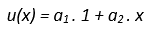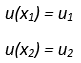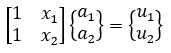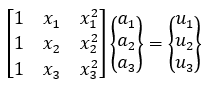top of page
Search

# What Are Shape Functions In FEA - And How Are They Derived?

Many of us have utilized the power of FEA solutions, such as Abaqus, to analyze all manner of products. But how much do we really know about exactly how it works? In this article, we will learn how to discretize a simple 1D domain into linear and quadratic elements and derive shape functions by approximating the unknown variables over the elements.

## Mathematical Modeling

As we know, any physical problem can be expressed in terms of a mathematical governing equation. These governing equations can be simple algebraic equations (heat conduction between two points) or complex differential and functional descriptions (spring-mass system, Navier-Stokes). The exact numerical solution to these governing equations cannot be always obtained using standard solution methods like substitutions and separable equations. Finite element analysis (FEA) is a procedure to obtain approximate numerical solution to governing equations. This is achieved by converting the mathematical model to set of solvable algebraic equations and attaining the numerical solution at set of discrete locations in the computational domain.

## Deriving Weak Form

Once the governing differential equation (strong form) is obtained by considering the physics, kinematics and dynamics of a physical problem, the weak form can be obtained using different approaches like virtual work principle and Galerkin weighted residual method. For example, the weak form of 1D elastic problem is given below,The figure below summarized different techniques for obtaining weak form equations.## Derivation of Shape Functions

The above weak form equation is solved in a discretized computational domain. The figure below shows the discretization of 1D domain into 3 elements.Let us assume the unknown displacement u at the nodes are u1, u2, u3 and u4. This unknown variable u is approximated using a polynomial function in x as given below,where 1, x, x2, x3 are called monomial basis functions and a1, a2, a3, a4 are coefficients.For linear elements, a linear function is assumed for u.Since there are two unknown coefficients, we need two boundary conditions to solve them.Substituting the boundary conditions in the linear approximation gives,This can be written in matric form as,The above equations are solved for unknown coefficients a1, and a2 which gives,where,Here N1(x), N2(x) are called linear shape functions.Similarly, if we have three node elements as shown in the below figure, a quadratic polynomial is assumed for u. Here, we will need three nodal displacements to solve for the unknown coefficients.The coefficients are obtained by solving the equations below,Expressing u in terms of unknown coefficients gives,where,Here N1(x), N2(x) and N3(x) are called quadratic shape functions.The shape functions are to be calculated for all the elements in the discretized domain. They are substituted back into the weak form governing equations to perform finite element approximation and to derive element matrices and vectors. The above procedure can be extended to derive shape functions for 2D, 3D linear and quadratic elements.## Properties of Shape Functions

1. At a node, the value of shape function is either 0 or 1.2. Summation of all the shape functions at any point is equal to 1.3. Continuity

Within the element boundary, the polynomial approximation for u is Ccontinuous. The continuity across the element boundaries depends on the polynomial approximation for the variable. For example, the variable has only C0 continuity across the linear and quadratic element boundaries (i.e., its first derivative is piecewise continuous).4. Completeness

This is an important requirement for shape functions to ensure the convergence of finite element approximate solution to the exact solution of governing equation.

## Final Thoughts

Shape functions are the backbone of finite element method as they play a crucial part in converting the weak form equation to set of computer solvable algebraic equations.

Hopefully this article has provided some basic understanding of discretization of a 1D computational domain and derivation of shape functions.

If you need help with FEA or simulation in general, don’t hesitate to give us a call! We’re passionate about what we do, and we would love to learn more about your unique engineering challenges.

15,065 views
bottom of page